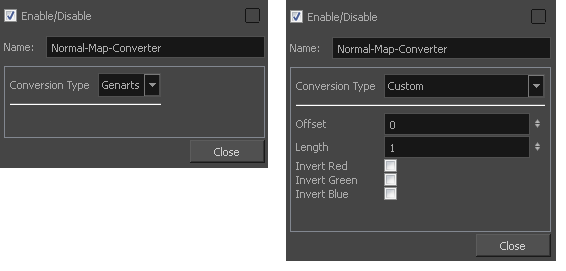# Normal Map Converter Node

The normal map will carve and emboss a flat plane using vectors. The X, Y and Z coordinates of the normal map vectors are stored in RGB values to convert the 3D aspect into a visual result and use it to create various effects, such as light reflections (light shading). The X value is stored in the Red channel. The Y value is stored in the Green channel. The Z value is stored in the Blue channel.

NOTE Note that not all normal map formats are supported.

The Normal Map Converter uses the following formula per channel:

• `value * (+/- length) + offset`
• `* +/- depending if inverse is set to true ("-" if true)`Parameter Description Conversion Type By default, the conversion type is set to Genarts. If you work with a different manufacturer and want to adjust the parameters, set the conversion type to Custom. Offset Offsets the original position of the vector. You need to read the plugin documentation to know the offset you need to use. You must use the same offset value as the manufacturer is using. By default the offset is set to 0. Length The Normal Map Converter normalizes Harmony vectors to 1. By default the length value is set to 1. If your plugin requires shorter or longer vectors, enter a value smaller or greater than 1. It will multiply the vector length. Invert Red Inverts the red value (x). If your value is 125, the invert setting will transform it to -125. Invert Green Inverts the green value (y). If your value is 125, the invert setting will transform it to -125. Invert Blue Inverts the blue value (z). If your value is 125, the invert setting will transform it to -125.